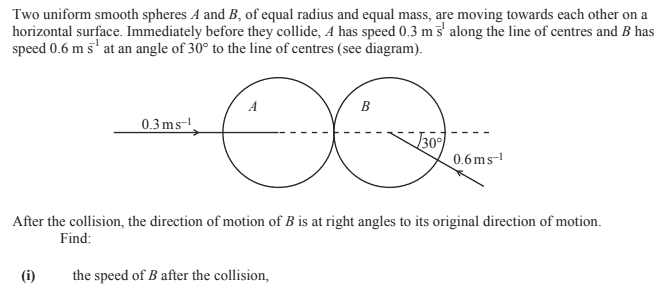# 2-D Momentum Problem -- Elastic collision of two spheres

• Jimmy87

#### Jimmy87

Homework Statement
Determining velocity after a 2-D impact.
Relevant Equations
p = mv
Hi,

Here is the problemWhat is required to answer this question is two assumptions. Firstly, the component of the momentum normal to the centre line is the same before and after. Therefore, secondly, A must recoil entirely in the horizontal plane. This is the only way to answer this question and I can get the answer (0.34 m/s which I know to be correct). How do we know after this collision A is confined to the horizontal plane? Thanks.

How do we know after this collision A is confined to the horizontal plane? Thanks
We don't. Snooker balls sometimes jump up off the table after a collision.

You just have to assume that doesn't happen in this case.

••BvU and MatinSAR
We don't. Snooker balls sometimes jump up off the table after a collision.

You just have to assume that doesn't happen in this case.
Ah sorry I wasn’t clear. The only way to answer this question is if ball A recoils along the centre line. How do we know it doesn’t have any vertical component after the collision (vertical in terms of the plane of the surface).

The only way to answer this question is if ball A recoils along the centre line. How do we know it doesn’t have any vertical component after the collision (vertical in terms of the plane of the surface).
The balls are "smooth". What is the direction of the force that B exerts on A?

•Jimmy87
How do we know it doesn’t have any vertical component after the collision (vertical in terms of the plane of the surface).
A better term is lateral or tangential, rather than vertical.

•Jimmy87
Ah sorry I wasn’t clear. The only way to answer this question is if ball A recoils along the centre line. How do we know it doesn’t have any vertical component after the collision (vertical in terms of the plane of the surface).
To acquire a vertical (tangential) component of momentum, a vertical force is needed during the collision. What entity could be the origin of that force? See post #4 by @TSny.

•Jimmy87
To acquire a vertical (tangential) component of momentum, a vertical force is needed during the collision. What entity could be the origin of that force? See post #4 by @TSny.
Yeh I’m still struggling to visualise it. Ball B changes direction by moving through an angle of 90 degrees yet ball doesn’t changes it angle at all.

If the velocity is at an angle of 30 degrees will there not be a component normal to the line imparted on ball A?

Ball A can only exert a force perpendicular to its surface which is the radial direction from the center of A to the point of the collision. This means that the velocity of ball B can change only in that radial direction. Draw a vector diagram of the momentum of ball B before and after the collision showing this.

Yeh I’m still struggling to visualise it. Ball B changes direction by moving through an angle of 90 degrees yet ball doesn’t changes it angle at all.

If the velocity is at an angle of 30 degrees will there not be a component normal to the line imparted on ball A?
It says "smooth" spheres in the question, which is introductory physics code for "no friction". In reality, there would be some friction between the surfaces and some exchange of lateral momentum. A super slow-motion film of the collision of two snooker balls would be interesting from this perspective. To see how much (if any!) lateral momentum is imparted to the object ball.

•nasu
Ball A can only exert a force perpendicular to its surface which is the radial direction from the center of A to the point of the collision. This means that the velocity of ball B can change only in that radial direction. Draw a vector diagram of the momentum of ball B before and after the collision showing this.
Thanks I think I can visualise it now. So it’s because they collide exactly edge on with no friction. I guess if ball B collided with some of its radius within ball A’s radius it would impart a force perpendicular to the centre line into A? Is that right? Thanks

Thanks I think I can visualise it now. So it’s because they collide exactly edge on with no friction. I guess if ball B collided with some of its radius within ball A’s radius it would impart a force perpendicular to the centre line into A? Is that right? Thanks
This problem makes the simplifying assumption that the spheres have a frictionless contact. If you want to take the next step, I'd look online for an experimental analysis of these sorts of collisions. Introductory physics is essential in teaching you the basics and getting experience with the mathematics, but it only takes you so far in terms of understanding the real world.

What is required to answer this question is two assumptions. Firstly, the component of the momentum normal to the centre line is the same before and after. Therefore, secondly, A must recoil entirely in the horizontal plane. This is the only way to answer this question and I can get the answer (0.34 m/s which I know to be correct). How do we know after this collision A is confined to the horizontal plane? Thanks.
What horizontal plane are you referring to?
The plane on which the balls are sliding or rolling on, or the one perpendicular to the shown picture and containing the trajectory of A?

Homework Statement:: Determining velocity after a 2-D impact.
Relevant Equations:: p = mv

0.34 m/s which I know to be correct
Should be 0.6 tan(30), which is nearer to 0.35, no?